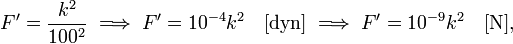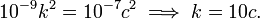# Statcoulomb

In physics, a statcoulomb (symbol statC), formerly known as esu of charge, is the unit of electric charge in the cgs-esu (centimeter-gram-second electrostatic system) of units.

By definition, a point charge has magnitude one statcoulomb if it repels a point charge of equal magnitude at a distance of 1 centimeter with a force of one dyne.

1 statC = 1 esu of charge = C/(10⋅c) ≈ 3.335 640 95⋅10−10 C

where C (coulomb) is the SI unit of charge and c is the SI speed of light (c ≈ 3⋅108 m/s).

## Conversion to coulomb

The conversion of statC to C can be done as follows. Write

1 C = k statC,

and compute k. Consider two charges of 1 C each, 1 m apart, then the force between them is according to Coulomb's law in SI units,$F = \frac{1}{4\pi \varepsilon_0} = 10^{-7} c^2\quad \textrm{[N]}$

(see electric constant for the SI value of ε0). The same system of two charges has in cgs units a force in dyne (1 dyn = 10−5 N):$F' = \frac{k^2}{100^2}\; \Longrightarrow\; F' = 10^{-4} k^2\quad\textrm{[dyn]}\;\Longrightarrow\; F' = 10^{-9}k^2\quad \textrm{[N]},$

where we applied Coulomb's law in electrostatic units. Hence$10^{-9}k^2 = 10^{-7} c^2 \;\Longrightarrow\;k = 10 c.$